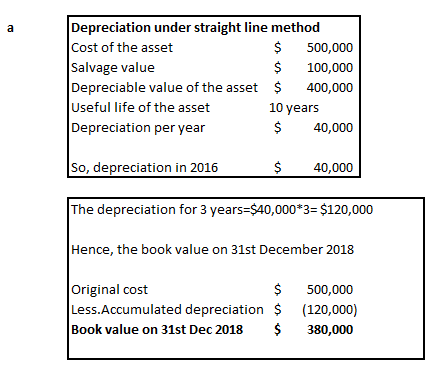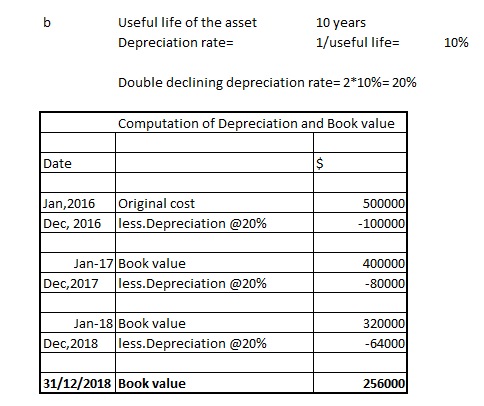Depreciation calculation methods Gandolfi Construction Co. purchased a used CAT 336DL earth mover at a cost of \$500,000 in January 2016. The company’s estimated useful life of this heavy equipment is 10 years, and the estimated salvage value is \$100,000.Required:a. Using straight-line depreciation, calculate the depreciation expense to be recognized for 2016, the first year of the equipment’s life, and calculate the equipment’s net book value at December 31, 2018, after the third year of the equipment’s life.b. Using declining-balance depreciation at twice the straight-line rate, calculate the depreciation expense to be recognized for 2018, the third year of the equipment’s life.

Question

Depreciation calculation methods Gandolfi Construction Co. purchased a used CAT 336DL earth mover at a cost of \$500,000 in January 2016. The company’s estimated useful life of this heavy equipment is 10 years, and the estimated salvage value is \$100,000.
Required:
a. Using straight-line depreciation, calculate the depreciation expense to be recognized for 2016, the first year of the equipment’s life, and calculate the equipment’s net book value at December 31, 2018, after the third year of the equipment’s life.
b. Using declining-balance depreciation at twice the straight-line rate, calculate the depreciation expense to be recognized for 2018, the third year of the equipment’s life.

Step 1

The depreciation under straight line method is computed by following formula (cost-salvage value)/estimated useful life. The depreciation is same throughout the useful life of the asset

In the declining balance method, the depreciation is computed on the remaining book value of the asset until it freaches the salvage value. So, the depreciation amount keeps declining over the years and the asset is depreciated more in the initial years

Step 2Step 3...

Want to see the full answer?

See Solution

Want to see this answer and more?

Our solutions are written by experts, many with advanced degrees, and available 24/7

See Solution
Tagged in